Categories

# Dirac Delta Function – δ(t)Figure 1 Properties of Unit Impulse

### Introduction

The unit impulse or Dirac Delta function δ(t) is an essential building block of modern telecommunications, similar in importance to the FFT that was examined in the previous post. The function came about as a result of research done by the British Physicist Paul Dirac on the modelling of a point charge (Ref.1). It is actually a so called distribution or a generalized function, and its properties can be examined in the limiting sense (Ref.2 p45). These can be visualized very effectively using telecom software such as Scicos or GNU Radio.

### Properties

The essential properties can be summarized by the following equations shown in Figure 1. The unit impulse is zero everywhere except at the origin where it has an infinite amplitude with an infinitely small duration such that the area remains 1. The Fourier Transform yields an infinite spectrum with unit value. In the real world this means that a very fast pulse, similar to a radar pulse, has a very wide flat spectrum.

### Limiting Behaviour

The unit impulse or Dirac Delta function δ(t) can be modeled by considering the Fourier Series of a Rectangular Pulse Train as shown in Figure 2. The unit impulse is the pulse train with an amplitude A = [1/duration] so that the area = A x duration = 1 and with T —> infinity & duration —> zero.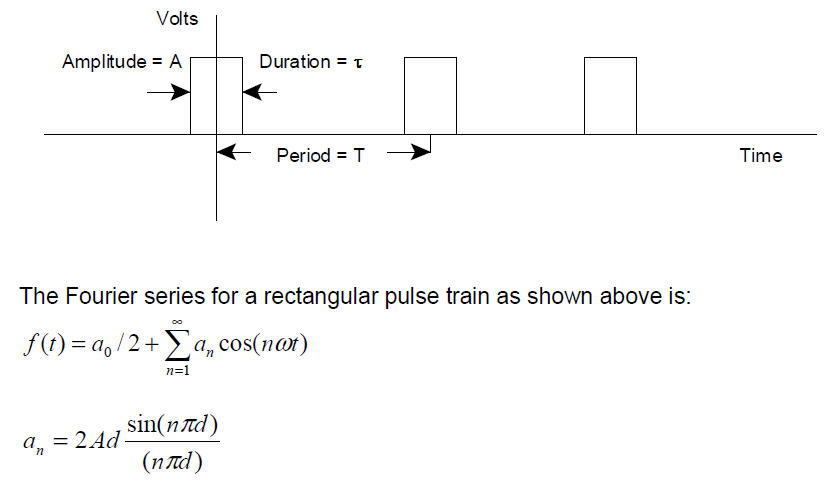Figure 2 Fourier Series Expansion of Rectangular Pulse Train

### Scicos Model Rectangular Pulse Train

Figure 3 shows a Scicos model that can be used to examine the unit impulse. We start off with an RPT Rectangular Pulse Train waveform of duration = 10msec and period of 100msec. Since this is a periodic waveform, it has Fourier components that are spaced by 1/period = 10Hz and spectral nulls at 1/pulse_width = 1/10msec = 100Hz. This is shown in Figure 4. If we now decrease the pulse width to 5%, the null moves out to 200Hz, and the components are still spaced by 10Hz. This is shown in Figure 5. In Figure 6, we increase the period to 200msec. Now the components are only spaced by 5Hz. This is shown in Figure 6.

The Unit Impulse is the RPT where the period goes to infinity, thus making the spacing between components infinitely small or a continuous spectrum. The amplitude goes to infinity and the duration goes to zero keeping the area =1. Since the duration goes to zero, the spectral null moves out to infinity. Thus we have the Fourier transform in the limit.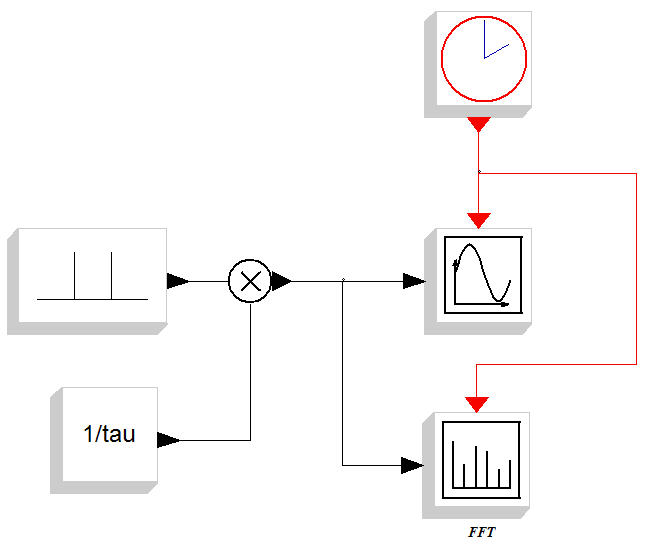Figure 3 Scicos Model of RPT Rectangular Pulse Train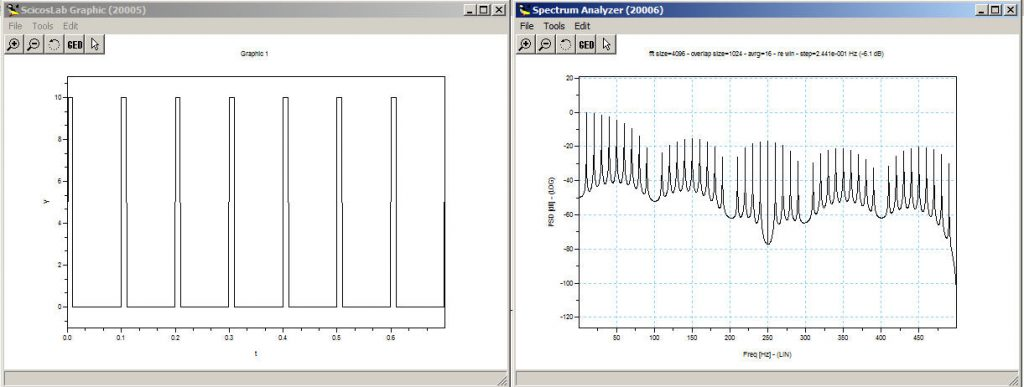Figure 4 RPT d=10%=10msec/T=100msec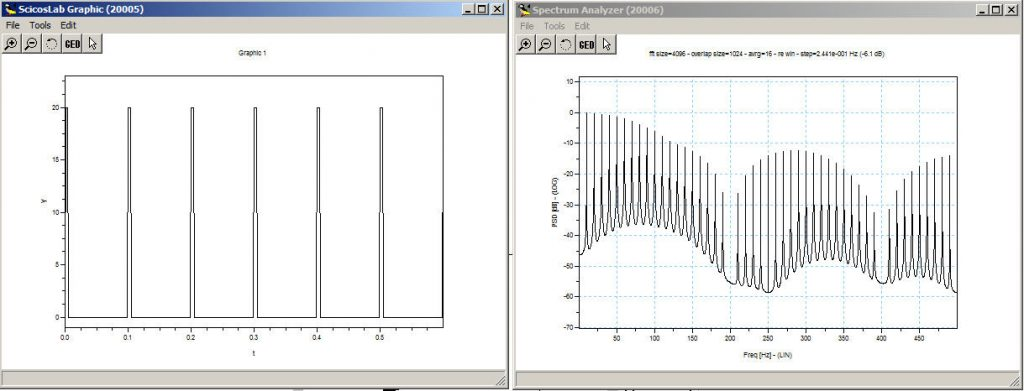Figure 5 RPT d=5%=5msec/T=100msec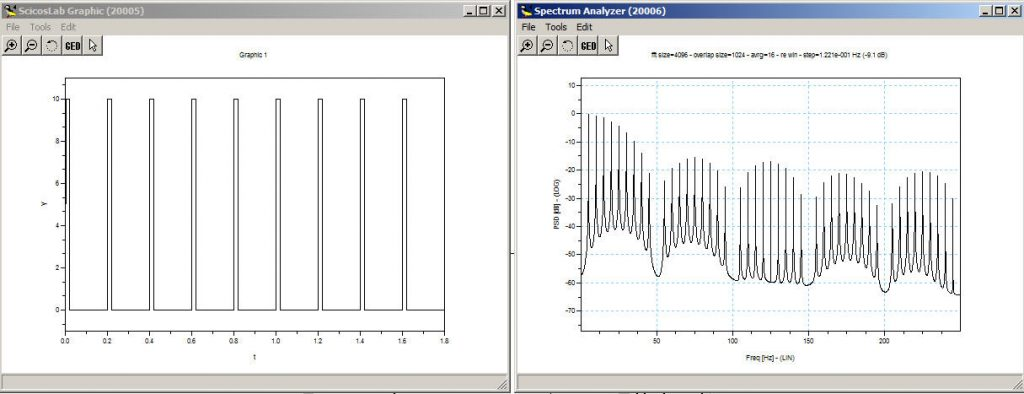Figure 6 RPT d=10%=20msec/T=200msecYouTube Channel

### References

#1 – “Dirac Delta Function”, Wikipedia

#2 – “Communication Systems: An Introduction to Signals and Noise in Electrical Communication”, A. Bruce Carlson, McGraw-Hill, 1968, Library of Congress 68-2276109955